Disclaimer: This is an example of a student written essay.

Any scientific information contained within this essay should not be treated as fact, this content is to be used for educational purposes only and may contain factual inaccuracies or be out of date.

# Preformulation Testing for Chemical Properties of Drug

 ✅ Paper Type: Free Essay ✅ Subject: Chemistry ✅ Wordcount: 4524 words ✅ Published: 30th Nov 2017

PREFORMULATION STUDIES

Preformulation testing involved investigation of physical and chemical properties of a drug substance alone and when combined with excipients. It was the first step in the rational development of dosage forms.

These studies are categorised as under:

1. API characterization

2. Drug-Excipient Compatibility study

API Characterization

• Organoleptic Evaluation

These are preliminary characteristics of any substance which is useful in identification of specific material. Following physical properties of API were studied.

a) Colour

b) Odour

Table no. : Characterization of API

 Test Observation Colour White Odour Odourless
• Particle size distribution

Sieve analysis:

The sieve analysis main concept is to know the different drug particles size in the sample. The standard sieves with larger pore size i.e., with less sieve number on the top position are placed one over the other and followed by sieves of decreasing pore size i.e., with larger sieve number towards the bottom.

Procedure:

1. Clean and dried sieves #40,#60,#80,#100,#120 and bottom meshes were collected
2. Individual weight of each sieve was noted.
3. These sieves were arranged in ascending order.
4. Weighed quantity of API was placed in #40 meshes.
5. Sieve shaker was set for 5 min at amplitude of 60.
6. Remove the setup from sieve shaker after 5minutes.
7. Each mesh was weighed individually and Calculate % retained in each size of mesh with following formula:

% retained = Final weight – initial weight x 100

Total weight taken

Table no. : Particle size distribution of API

 Sieve number Percentage of sample retained (%) Cumulative percentage of sample retained (%) 40 3.0 3.0 60 19.2 22.2 80 26.3 48.5 100 24.2 72.7 140 8.1 80.8 200 19.2 100.0
• pH-Solubility Profile:

The solubility studies for the drug were carried out using the orbital shaker. Solubility of the drug across different buffers was studied. The pH ranged from 1.2 to 6.8 (1.2 , 4.5, 6.8, and water). All the buffers were prepared according to USP 34 NF 29, 2011. Excess drug was added to 100 ml of water in stoppered conical flasks and were agitated continuously in a orbital shaker for 24 hrs at 200 rpm and room temperature (25° C), till saturation was observed. Then, the samples were filtered using 0.45 µ Nylon (47 mm) syringe filters. Now the filtered samples were analyzed using UV spectrophotometer Table No.6.3 below describes the procedure of buffers preparation.

Table 6.5 preparation of buffers

 Buffer Procedure PH 1.2 buffer 8.5 ml of Conc. HCL was added to 1000 mL volumetric flask. Then it was diluted and made up to volume with water PH 4.5 phosphate buffer 13.61 gm of KH2PO4 was added to 1000 mlvolumetric flask. Then it was made up to volumewith water PH 6.8 phosphate buffer 250 mL of 0.2 M monobasic potassium phosphate solution was taken in a 1000 mL volumetric flask. Then 112 mL 0.2M Sodium hydroxide solution was added to it and water was added to make up to the volume

Table7.3 Solubility of API in buffers of different pHs

 PH Solubility(mg/ml) 1.2 42.36 4.5 44.96 6.8 0.80 Water 0.674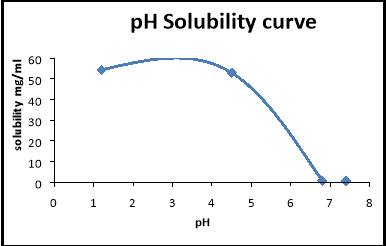Fig 7.1 pH Solubility curve of API

Drug excipient compatibility study

There is always possibility of Drug – excipient interaction in any formulation due to their intimate contact. It is also necessary to determine any possible interaction between excipients used in the formulation. This will also indicate success of stability studies.

• Preliminary studies:

Method: Physical observation

Condition: 40 ±2 o C and 75 ± 5% RH

Procedure:

• Drug was mixed with excipients in 1: 1 ratios as indicated in the Table 6.6
• These mixtures were kept in a 5ml glass vials and packed properly.
• In dry close method glass vials are closed with rubber stoppers
• These vials are exposed to 25ËšC /60 % RH & 40ËšC /75 % RH.
• Blend (1gm) was prepared and filled in vials. Observations for physical appearance were made at the end 4 weeks.
 S.No EXCIPIENTS DRUG: EXCIPIENT RATIO 1 Polyethyleneoxide 1:1 2 HPMCK100M 1:1 3 MCC 1:1 4 Cellulose acetate 1:1 5 Sodiumchloride 1:1 6 Citric acid 1:1 7 Sodium lauryl sulphate 1:1 8 Magnesium stearate 1:1 9 Talc 1:1

Table 7.4 Results of Drug-Excipient compatibility at 25oC/60% RH

 S.no: Excipent Colour change Lumps Caking O C O C O C 1 Polyethylene oxide X X X X X X 2 HPMC X X X X X X 3 MCC X X X X X X 4 Cellulose acetate X X X X X X 5 Sodium chloride X X Lumps observed X X X 6 Citric acid X X Lumps observed X X X 7 Sodium lauryl sulphate X X Lumps observed X X X 7 Magnesium stearate X X X X X X 8 Talc X X X X X X

Note: x – indicates no change, O- open condition ,C- close condition

Table 7.5 Results of Drug-Excipient compatibility at 40oC / 75% RH

 S.no: Excipient Colour change Lumps Caking O C O C O C 1 Poly ethylene oxide X X X X X X 2 HPMC X X X X X X 3 MCC X X X X X X 4 Cellulose acetate X X X X X X 5 Sodium chloride X X X X Caking observed X 6 Citric acid X X X X Caking Observed X 7 Sodium lauryl sulphate X X X X Caking observed X 8 Magnesium stearate X X X X X X 9 Talc X X X X X X
• FTIR Study

FTIR study:

FTIR studies were carried out for pure drug alone and blend of drug & excepients. The FTIR spectroscopy (BRUKER Optics FTIR spectrophotometer) is employed as analytical tool to check the drug-excepients interaction, using the KBr disc method. The FTIR spectra were scanned and recorded between 400 and 4000 cm-1

Method:

Nearly to a fine alkali halide (example KBr) powder of 200 to 250 mg 0.1 to 1.0 % sample is mixed well. Later it is pulverized and in a pellet-forming die it is placed. Around an 8 tons force under a vacuum of several mm Hg is applied to form transparent pellets.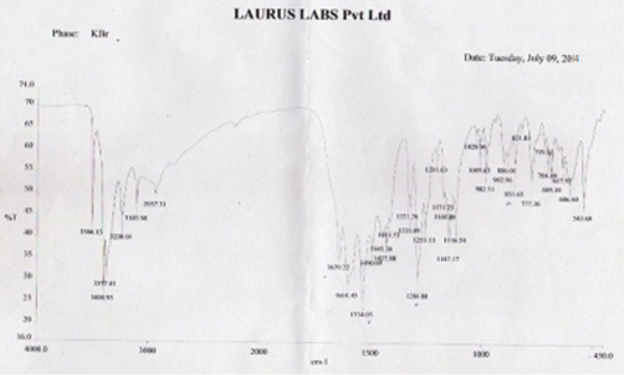FTIR spectroscopy of pure drug of Famotidine

 S.no Type of vibration Actual frequency (cm-1) Observed frequency (cm-1) Confirmation

Table no. : Interpretation of FTIR spectra of pure famotidineFTIR spectroscopy of drug and excipient blends

Table 7.6 Peaks of FTIR study

 Peaks ( cm –1) Functional groups 3506.13 –OH 3377.41-3400.95 –NH2 3238.03 -NH 1445.38- 1639.22 C=N 689.10 -606.6 C-S 1320.81 S(=O) 2 asymmetric stretching 1147.17 S(=O) 2 symmetric stretching

Table no. : Interpretation of FTIR spectra of pure famotidine

 S.no Type of vibration Actual frequency (cm-1) Observed frequency (cm-1) Confirmation

ANALYTICAL METHOD

ESTIMATION OF FAMOTIDINE:

A solution of Famotidine was prepared in 0.1 N HCl and Phosphate buffer pH 4.5and 6.8 UV spectrum was taken using Perkin Elmer UV/Vis double beam spectrophotometer.The UV maxima of Famotidine was found to be 265 nm in both 0.1N HCl & pH 4.5. In pH 6.8 it was found to be 268 nm

• Preparation of standard curve of famotidine in 0.1N HCL & pH 4.5 phosphate buffer:

100 mg Famotidine each was dissolved in 0.1 N HCl and pH 4.5 buffer and volume is made up to 100 with respective buffer. 10 mL of stock solution (1mg/ml) was further diluted upto 100 ml with respective buffer to obtained solution of 100 µg/mL.Now from stock 2 further dilutions were done with respective buffer to obtain solutions of 2, 5, 10, 15, 20 and 25 µg/ml Absorbance of each solution was measured at 265 nm using Perkin Elmer UV/Vis double beam Spectrophotometer.

• Preparation of standard curve in ph 6.8 phosphate buffer:

10 mg Famotidine each was dissolved in pH 6.8 phosphate buffer and volume is made

up to 100 ml to obtain solution of 100 µg/ml. Now from this stock solution further dilutions were done with PH 6.8 to obtain solutions of 10 , 20 , 30 and 40 µg/ml Absorbance of each solution was measured at 268 nm using Perkin Elmer UV/Vis double beam Spectrophotometer.

The experiment was performed in triplicate and based on average absorbance; the equation for the best line was generated. The results of standard curve prepared in pH 1.2, 4.5 & 6.8 were shown below

Table 7.7 Standard curve of API in PH 1.2 , 4.5 & 6.8 buffers

 Concentration Absorbance in pH 1.2 Absorbance in pH 4.5 Absorbance in pH 6.8 2 0.085 0.082 – 4 0.141 0.148 – 5 0.189 0.186 – 10 0.333 0.341 0.251 15 0.510 0.497 – 20 0.701 0.651 0.467 25 0.852 0.806 – 30 – – 0.746 40 – – 0.989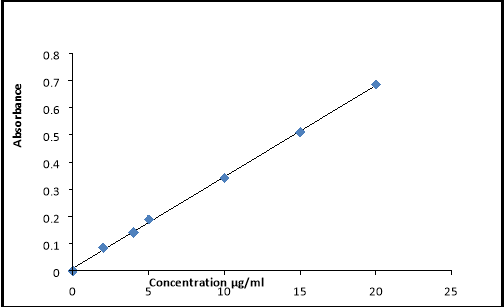FIG 7.4 standard curve at PH 1.2 Buffer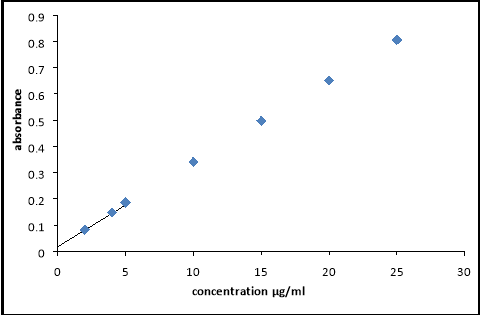Fig 7.5 standard curve at PH 4.5 Buffer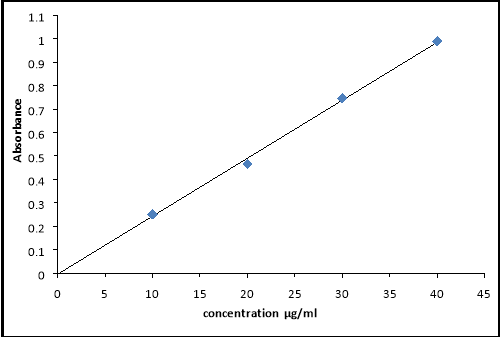Fig 7.6 standard curve at PH 6.8 Buffer

Calculation of initial dose and maintenance dose for the design of elementary osmotic pump of famotidine for 12 hours:

There are no sustained release formulations for famotidine in the market, hence the total dose (DT) consisting of initial (DI) and maintenance doses (DM) for formulating the famotidine sustained release was calculated as per Robinson and Eriksen equation with a zero order release principle36 . In this profile the rate of delivery is independent of the amount of drug remaining in the dosage form and constant over time as shown by the Eq. 6.1

Drug availability rate k0 = Rate in = Rate out Eq. 6.1

Where, k0 is the zero order rate constant for drug release (amount per time).

DI is required to give initial rapid release of drug so as to attain the minimum therapeutic level immediately after dosing.Inital dose (DI) = CSSAVG Vd Eq. 6.2

F

Where, C ssavg is the average steady state plasma level, V d is the volume of distribution and F is the fraction of dose absorbed.

k0 = DIKel Eq. 6.3

Where, Kel is overall first order drug elimination rate constant (per hour). Hence k 0 should be equal to the elimination rate constant so as to maintain the steady state condition.

In general the total dose required (D T) is the sum of maintenance dose (DM) and the initial dose (DI)

DT = D I + D M Eq. 6.4

In practice, D M (mg) is released over a period of time and is equal to the product of H (the number of hours for which sustained action is desired after initial dose) and the zero order rate constant, k0 (mg/hr).

Therefore the Eq. 6.4 can be expressed as

DT = D I + k0H Eq. 6.5

Ideally the maintenance dose (DM) is released after DI has produced a minimum therapeutic blood level of the drug. However due to the limits of formulations, drug release even starts from DM also from the beginning i.e. at t=0, thus increasing the initial drug level in the blood. Hence it is necessary to reduce the initial dose of the drug to account for the excess release for drug from DM by using a correction factor, k0tp. This correction factor is the amount of drug provided by DM during the period from t=0 to the time of the peak drug level, tp. The corrected initial dose (DI*) becomes DI-(k0tp). Then the total dose is

DT = DI* + k0H = (D I – k0tp) + k0H Eq. 6.6

• Pharmacokinetic parameters of famotidine:

Elimination half life (t1/2) of famotidine is 3 hrs (average of 2.5 to 3.5 hrs), the time to reach peak plasma (t p) is 3 hrs and Vd = 80.5 L and F = 0.4 54,55 . From the literature of the PEPCID (innovator product of famotidine in USA) label and pharmacological review information 49,, it was found that the plasma levels after multiple doses are similar to those after single doses indicating the C max is similar to Cssavg , therefore Cmax of 0.07 mg/L was taken as C ssavg .

• Calculation of D I and DM:

The initial dose (DI), corrected initial dose (DI*), maintenance dose (DM) and total dose (DT) were calculated according to calculations described above.

• Calculation of elimination rate constant:

Elimination rate constant (K el ) = 0.693/t 1/2

= 0.693/3 = 0.231 hr -1

• Calculation of initial dose:Inital dose (DI) = CSSAVG Vd Eq. 6.2

F = (0.07 X 80.5)/0.4

= 14.0875 mg

• Calculation of desired input rate (k 0):

Desired input rate from maintenance dose (k 0) = DIKel

= 14.0875 X 0.231 = 3.25 mg/hr

• Calculation of maintenance dose:

Maintenance dose (DM) = k0H (Since, H = the number of hours for which sustained action is desired after initial dose = (12-1) = 11 hrs)

= 3.25 X 11 = 35.796 mg

• Calculation of corrected initial dose DI*:

DI* = DI – (k0tp)

= 14.087 – (3.25 X 3 ) = 4.93 mg

• Calculation of total dose:

Total dose (D T) = D I* + D M

= 4.93 + 35.796 = 40.726 mg

From the above calculations the total dose obtained for sustained release of famotidine for 12 hrs is 40.726 mg. The total dose was rounded off to 40mg for the convenience. Initially the dosage form should release the total initial dose (i.e. 4.93 mg ~ 5.0 of drug, means 11% of total 50 mg dose) in the first 1 hr followed by maintenance dose (i.e. 40-5=35 mg of drug) for up to 12 hrs there after at a release rate of 3.25 mg/hr (i.e. 8.125% of total 40 mg dose). Based on these assumptions the theoretical release profile was predicted and shown

Table 6.7 Predicted theoretical release profile

 Time (hrs) % CDD 1 11.4 2 19.425 3 27.45 4 35.475 5 43.5 6 51.525 7 59.55 8 67.575 10 83.625 12 99.675

JNTUA-OTRI, Ananthapuramu 1

View all

## DMCA / Removal Request

If you are the original writer of this essay and no longer wish to have your work published on UKEssays.com then please:

Related Services

Related Lectures